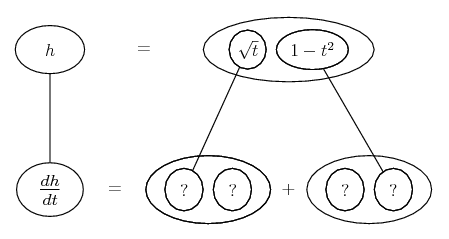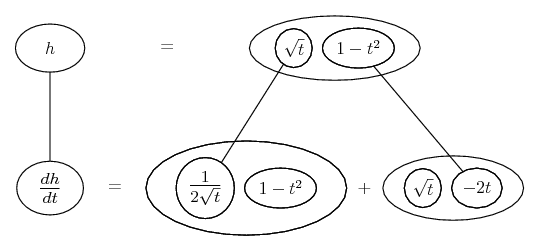# Thread: product rule

1. ## product rule

h(t) = [sqrt(t) ]*[(1-t²)]

find derivative using product rule any help on how to solve this would be nice i cant come to the awnser in the back of my book :/

also how do u do squareroot and other signs i see most people use when posting on forums?

2. Just in case a picture helps...... where... is the product rule. Straight continuous lines differentiate downwards (integrate up) with respect to t

Spoiler:__________________________________________

Don't integrate - balloontegrate!

Balloon Calculus; standard integrals, derivatives and methods

Balloon Calculus Drawing with LaTeX and Asymptote!

3.Originally Posted by maybnxtseasnh(t) = [sqrt(t) ]*[(1-t²)]

find derivative using product rule any help on how to solve this would be nice i cant come to the awnser in the back of my book :/

also how do u do squareroot and other signs i see most people use when posting on forums?
$\displaystyle h(t)=\sqrt{t}(1-t^2)$

Taking $\displaystyle f(t)=\sqrt{t}$ and $\displaystyle g(t) = (1-t^2)$

$\displaystyle f'(t) = \frac{1}{2\sqrt{t}}$ and $\displaystyle g'(t) = -2t$

$\displaystyle h'(t) = f(t)g'(t) + g(t)f'(t)$

$\displaystyle h'(t) = -2t\sqrt(t) + \frac{(1-t^2)}{2\sqrt{t}}$

If simplified,

$\displaystyle h'(t) = \frac{(1-t^2)}{2\sqrt{t}} - 2t^{\frac{3}{2}}$

4.Originally Posted by maybnxtseasnalso how do u do squareroot and other signs i see most people use when posting on forums?
$$\sqrt{t}\left(1-t^2\right)$$ gives $\displaystyle \sqrt{t}\left(1-t^2\right)$
You want to learn to use LaTeX

#### Search Tags

product, rule